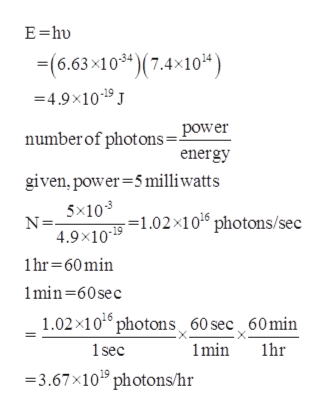# Answer the following questions about a Blu-ray laser:(a) The laser on a Blu-ray player has a wavelength of 405 nm. In what region of the electromagnetic spectrum is this radiation? What is its frequency?(b) A Blu-ray laser has a power of 5 milliwatts (1 watt = 1 J s−1). How many photons of light are produced by the laser in 1 hour?(c) The ideal resolution of a player using a laser (such as a Blu-ray player), which determines how close together data can be stored on a compact disk, is determined using the following formula: Resolution = 0.60(λ/NA), where λis the wavelength of the laser and NA is the numerical aperture. Numerical aperture is a measure of the size of the spot of light on the disk; the larger the NA, the smaller the spot. In a typical Blu-ray system, NA = 0.95. If the 405-nm laser is used in a Blu-ray player, what is the closest that information can be stored on a Blu-ray disk?(d) The data density of a Blu-ray disk using a 405-nm laser is 1.5 × 107 bits mm−2. Disks have an outside diameter of 120 mm and a hole of 15-mm diameter. How many data bits can be contained on the disk? If a Blu-ray disk can hold 9,400,000 pages of text, how many data bits are needed for a typed page? (Hint: Determine the area of the disk that is available to hold data. The area inside a circle is given by A = πr2, where the radius r is one-half of the diameter.)

Question
488 views

(a) The laser on a Blu-ray player has a wavelength of 405 nm. In what region of the electromagnetic spectrum is this radiation? What is its frequency?

(b) A Blu-ray laser has a power of 5 milliwatts (1 watt = 1 J s−1). How many photons of light are produced by the laser in 1 hour?

(c) The ideal resolution of a player using a laser (such as a Blu-ray player), which determines how close together data can be stored on a compact disk, is determined using the following formula: Resolution = 0.60(λ/NA), where λis the wavelength of the laser and NA is the numerical aperture. Numerical aperture is a measure of the size of the spot of light on the disk; the larger the NA, the smaller the spot. In a typical Blu-ray system, NA = 0.95. If the 405-nm laser is used in a Blu-ray player, what is the closest that information can be stored on a Blu-ray disk?

(d) The data density of a Blu-ray disk using a 405-nm laser is 1.5 × 107 bits mm−2. Disks have an outside diameter of 120 mm and a hole of 15-mm diameter. How many data bits can be contained on the disk? If a Blu-ray disk can hold 9,400,000 pages of text, how many data bits are needed for a typed page? (Hint: Determine the area of the disk that is available to hold data. The area inside a circle is given by A = πr2, where the radius r is one-half of the diameter.)

check_circle

Step 1

Hi, since there are multiple questions posted we will provide you with answers for the first three questions. Thank you.

Step 2

(a)The electromagnetic spectrum of the given radiation is in visible light region.

The frequency can be calculated as

Step 3

(b)The number of photons of...help_outlineImage TranscriptioncloseE hu -(6.63-10)(7.4 10*) -4.9x109 J power number of photons energy given, power 5 milliwatts 5x10 N= 4.9x1019 -=1.02 x1 0 photons/sec 1hr 60min 1min 60sec 1.02x106 photons 60 sec 60min 1sec min lhr 3.67x10 photons/hr fullscreen

### Want to see the full answer?

See Solution

#### Want to see this answer and more?

Solutions are written by subject experts who are available 24/7. Questions are typically answered within 1 hour.*

See Solution
*Response times may vary by subject and question.
Tagged in

### Physical Chemistry